Next: 4.2.2.2 Dirichlet Type Boundary Up: 4.2.2 Boundary Models Previous: 4.2.2 Boundary Models

### 4.2.2.1 Boundary Flux Equation System

Under the assumption of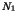and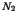quantities on the segments 1 and 2, respectively, (4.7) and (4.8) represent a linear equation system for the partial current densities of all involved quantities crossing the interface in normal direction. In these equations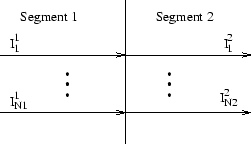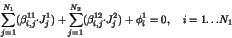(4.7)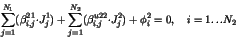(4.8)

All coefficients,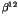,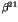, and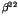are functions of the simulation time, the process temperature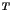, and the spatial coordinatesand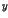. The coefficients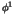andmay depend on the concentrations of the quantities on both sides of the interface. The model developer has to provide MDL classes which determine the actual values of the coefficients and their derivatives to the quantity concentrations which are the dependent variables in the PROMIS-NT internal equation system.Next: 4.2.2.2 Dirichlet Type Boundary Up: 4.2.2 Boundary Models Previous: 4.2.2 Boundary Models
Robert Mlekus
1999-11-14